Скачать презентацию Econ 101 Microeconomics Chapter 6 Production and Cost

f6240859698f608fac5451abde96fe6c.ppt

• Количество слайдов: 34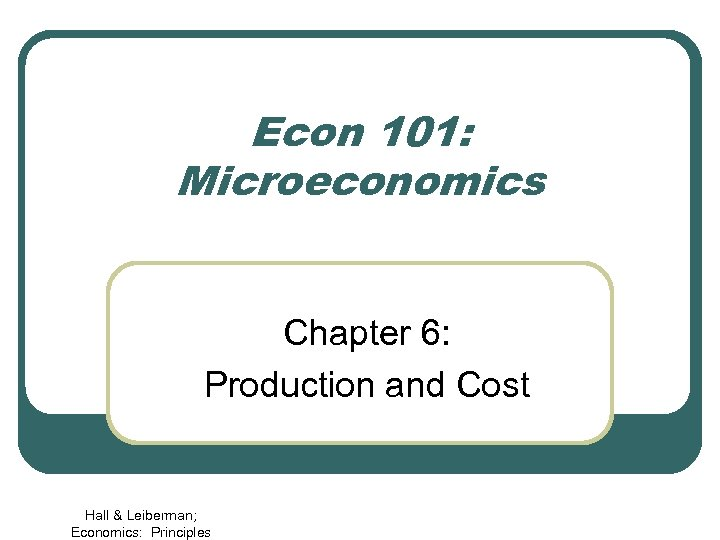Econ 101: Microeconomics Chapter 6: Production and Cost Hall & Leiberman; Economics: Principles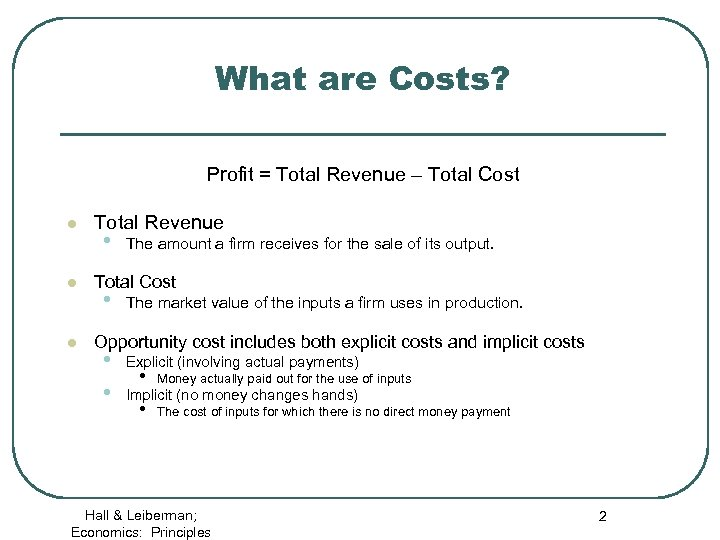What are Costs? Profit = Total Revenue – Total Cost l l l Total Revenue • The amount a firm receives for the sale of its output. Total Cost • The market value of the inputs a firm uses in production. Opportunity cost includes both explicit costs and implicit costs • • Explicit (involving actual payments) • Money actually paid out for the use of inputs • The cost of inputs for which there is no direct money payment Implicit (no money changes hands) Hall & Leiberman; Economics: Principles 2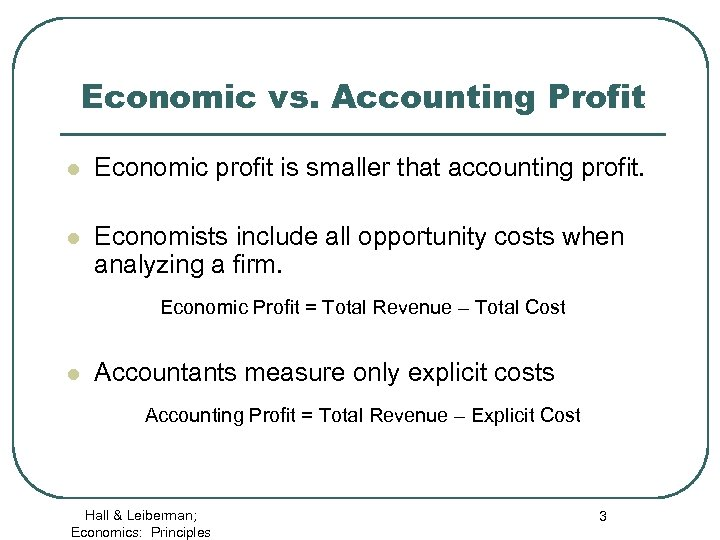Economic vs. Accounting Profit l Economic profit is smaller that accounting profit. l Economists include all opportunity costs when analyzing a firm. Economic Profit = Total Revenue – Total Cost l Accountants measure only explicit costs Accounting Profit = Total Revenue – Explicit Cost Hall & Leiberman; Economics: Principles 3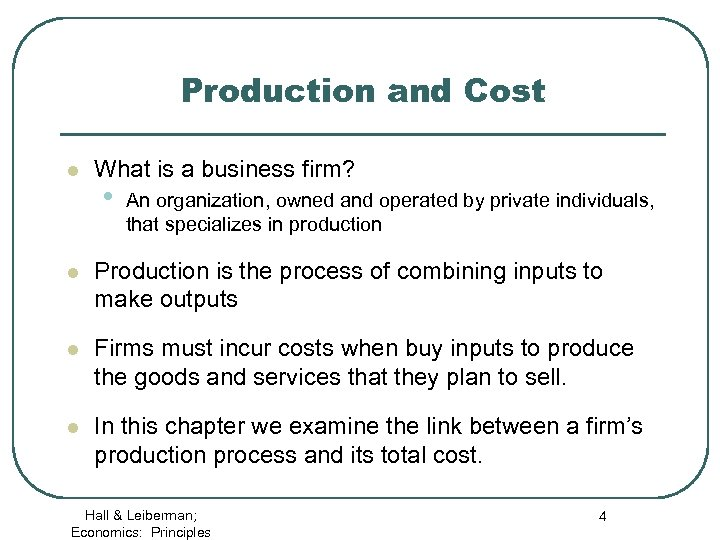Production and Cost l What is a business firm? • An organization, owned and operated by private individuals, that specializes in production l Production is the process of combining inputs to make outputs l Firms must incur costs when buy inputs to produce the goods and services that they plan to sell. l In this chapter we examine the link between a firm’s production process and its total cost. Hall & Leiberman; Economics: Principles 4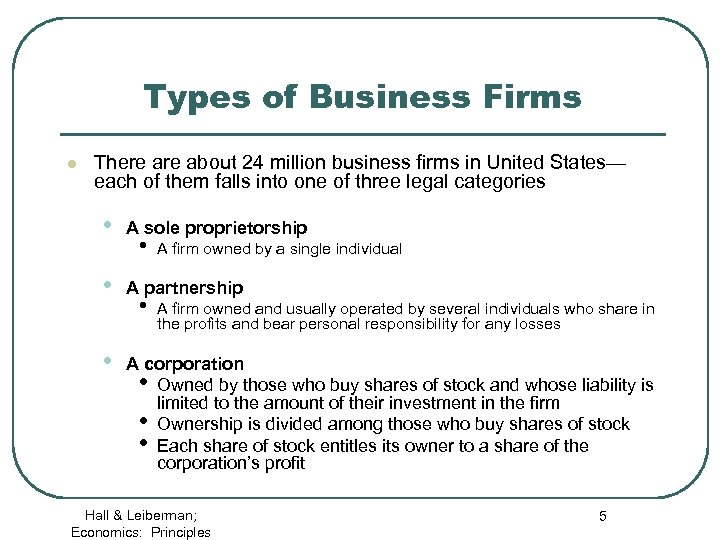Types of Business Firms l There about 24 million business firms in United States— each of them falls into one of three legal categories • A sole proprietorship • A partnership • A corporation • Owned by those who buy shares of stock and whose liability is limited to the amount of their investment in the firm • Ownership is divided among those who buy shares of stock • Each share of stock entitles its owner to a share of the corporation’s profit • • A firm owned by a single individual A firm owned and usually operated by several individuals who share in the profits and bear personal responsibility for any losses Hall & Leiberman; Economics: Principles 5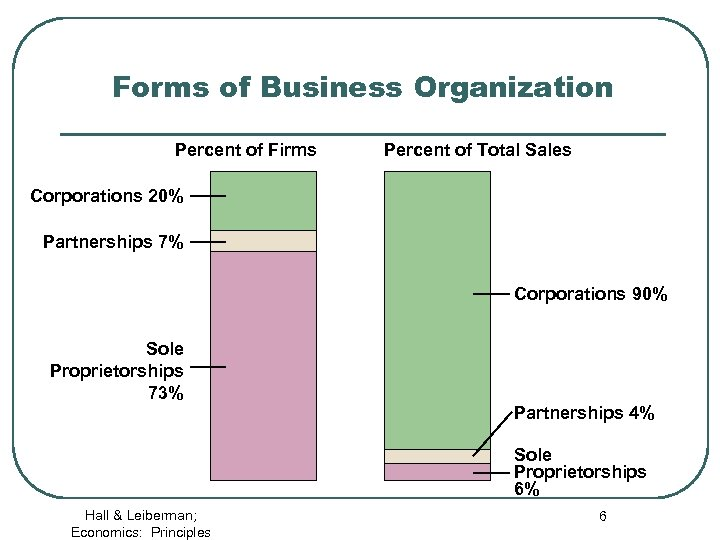Forms of Business Organization Percent of Firms Percent of Total Sales Corporations 20% Partnerships 7% Corporations 90% Sole Proprietorships 73% Partnerships 4% Sole Proprietorships 6% Hall & Leiberman; Economics: Principles 6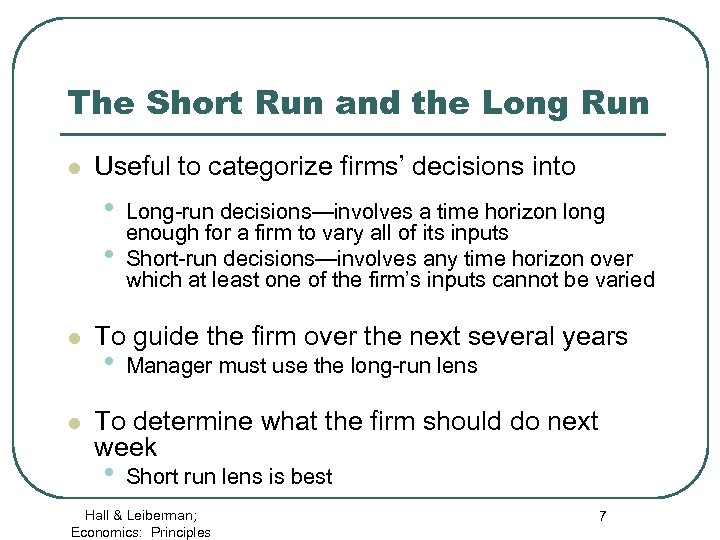The Short Run and the Long Run l Useful to categorize firms’ decisions into • • l l Long-run decisions—involves a time horizon long enough for a firm to vary all of its inputs Short-run decisions—involves any time horizon over which at least one of the firm’s inputs cannot be varied To guide the firm over the next several years • Manager must use the long-run lens To determine what the firm should do next week • Short run lens is best Hall & Leiberman; Economics: Principles 7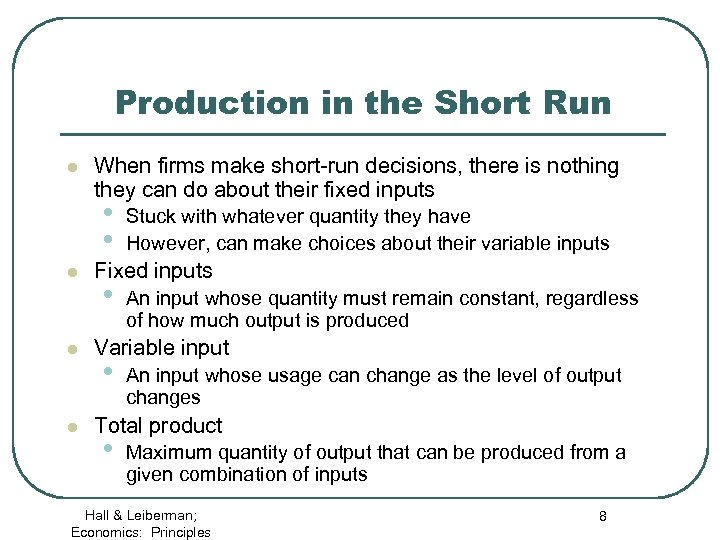Production in the Short Run l When firms make short-run decisions, there is nothing they can do about their fixed inputs • • l l l Stuck with whatever quantity they have However, can make choices about their variable inputs Fixed inputs • An input whose quantity must remain constant, regardless of how much output is produced Variable input • An input whose usage can change as the level of output changes Total product • Maximum quantity of output that can be produced from a given combination of inputs Hall & Leiberman; Economics: Principles 8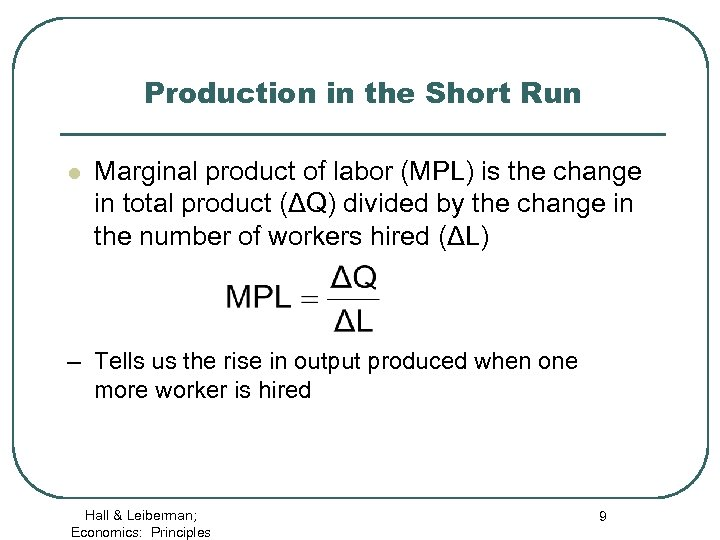Production in the Short Run l Marginal product of labor (MPL) is the change in total product (ΔQ) divided by the change in the number of workers hired (ΔL) – Tells us the rise in output produced when one more worker is hired Hall & Leiberman; Economics: Principles 9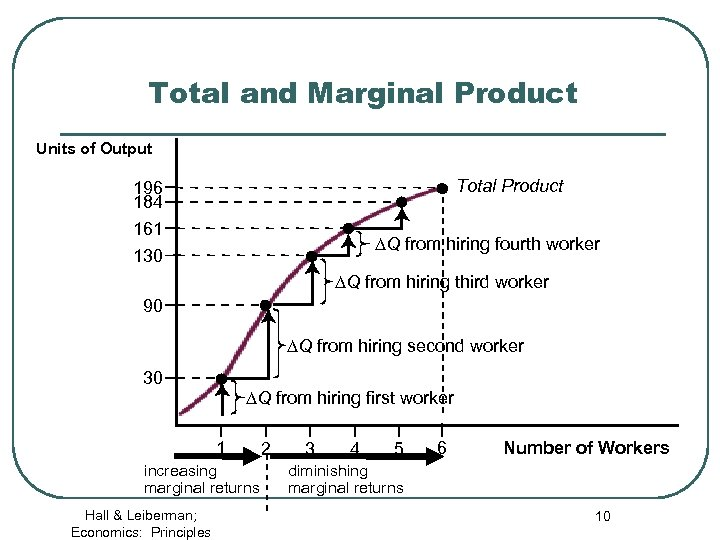Total and Marginal Product Units of Output Total Product 196 184 161 DQ from hiring fourth worker 130 DQ from hiring third worker 90 DQ from hiring second worker 30 DQ from hiring first worker 1 increasing marginal returns Hall & Leiberman; Economics: Principles 2 3 4 5 6 Number of Workers diminishing marginal returns 10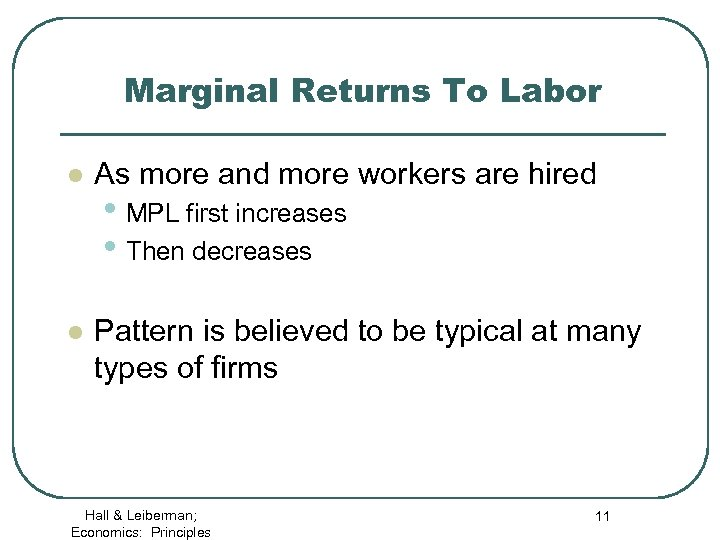Marginal Returns To Labor l As more and more workers are hired l Pattern is believed to be typical at many types of firms • MPL first increases • Then decreases Hall & Leiberman; Economics: Principles 11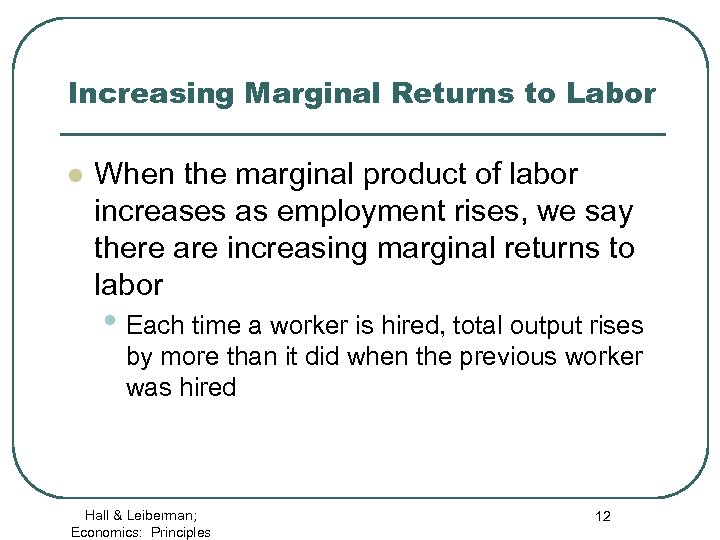Increasing Marginal Returns to Labor l When the marginal product of labor increases as employment rises, we say there are increasing marginal returns to labor • Each time a worker is hired, total output rises by more than it did when the previous worker was hired Hall & Leiberman; Economics: Principles 12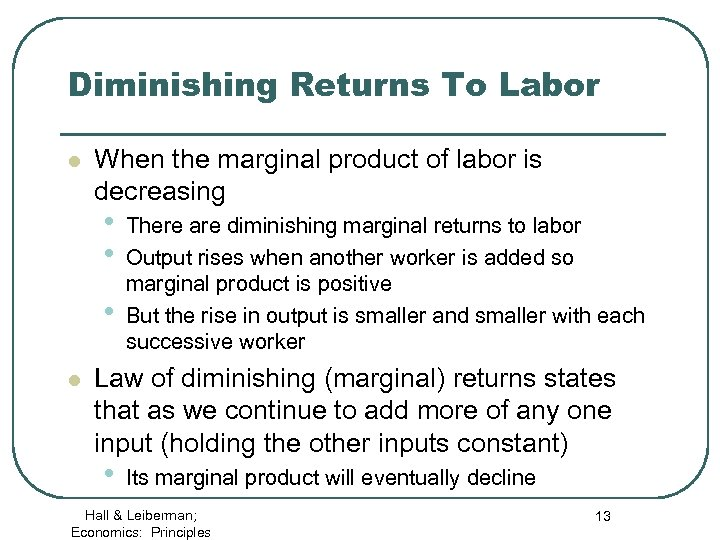Diminishing Returns To Labor l When the marginal product of labor is decreasing • • • l There are diminishing marginal returns to labor Output rises when another worker is added so marginal product is positive But the rise in output is smaller and smaller with each successive worker Law of diminishing (marginal) returns states that as we continue to add more of any one input (holding the other inputs constant) • Its marginal product will eventually decline Hall & Leiberman; Economics: Principles 13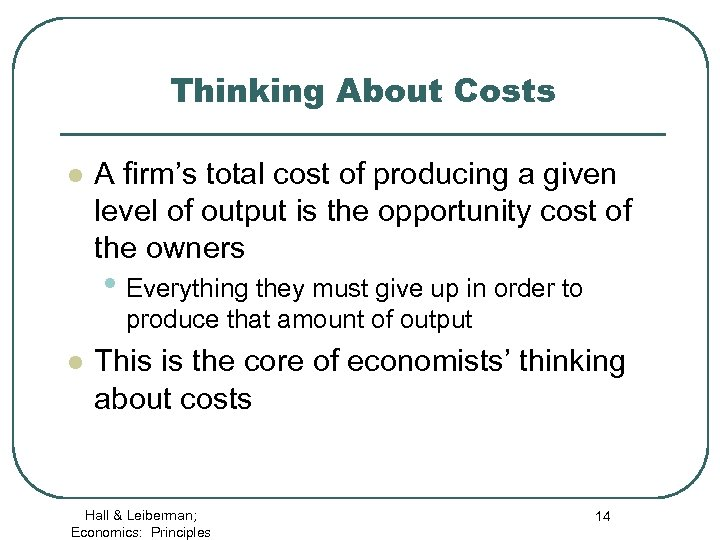Thinking About Costs l A firm’s total cost of producing a given level of output is the opportunity cost of the owners • Everything they must give up in order to produce that amount of output l This is the core of economists’ thinking about costs Hall & Leiberman; Economics: Principles 14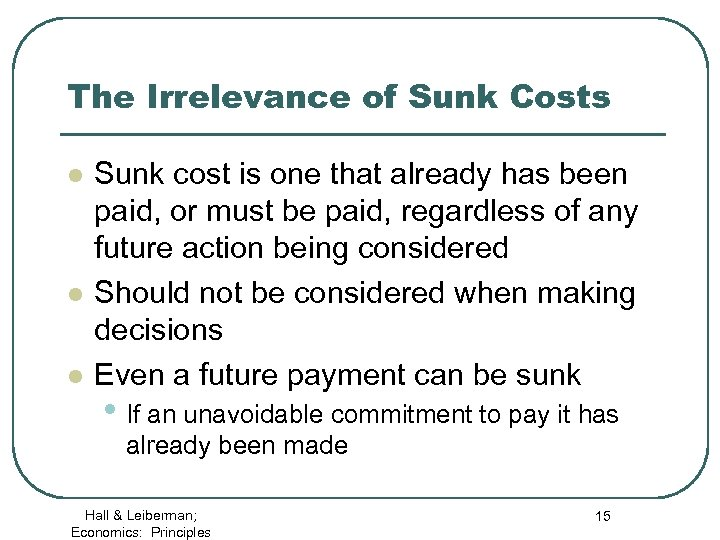The Irrelevance of Sunk Costs l l l Sunk cost is one that already has been paid, or must be paid, regardless of any future action being considered Should not be considered when making decisions Even a future payment can be sunk • If an unavoidable commitment to pay it has already been made Hall & Leiberman; Economics: Principles 15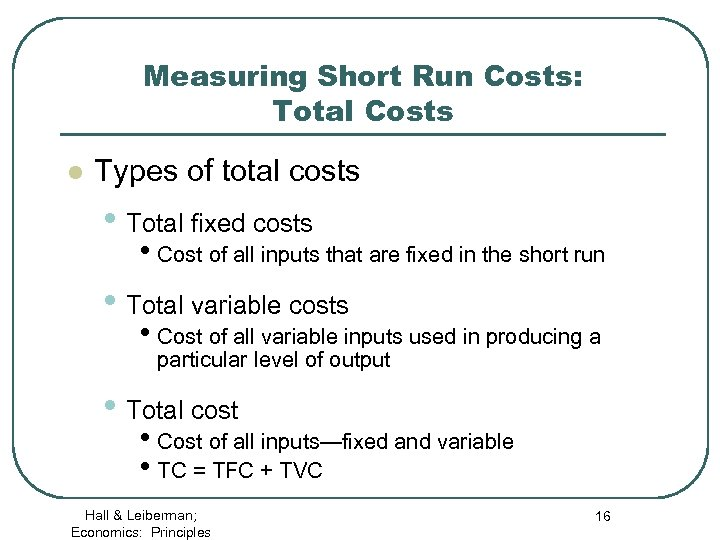Measuring Short Run Costs: Total Costs l Types of total costs • Total fixed costs • Cost of all inputs that are fixed in the short run • Total variable costs • Cost of all variable inputs used in producing a particular level of output • Total cost • Cost of all inputs—fixed and variable • TC = TFC + TVC Hall & Leiberman; Economics: Principles 16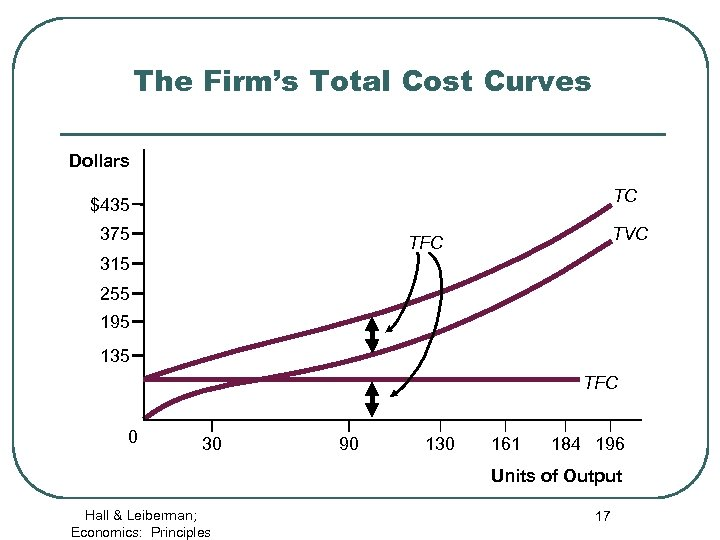The Firm’s Total Cost Curves Dollars TC \$435 375 TVC TFC 315 255 195 135 TFC 0 30 90 130 161 184 196 Units of Output Hall & Leiberman; Economics: Principles 17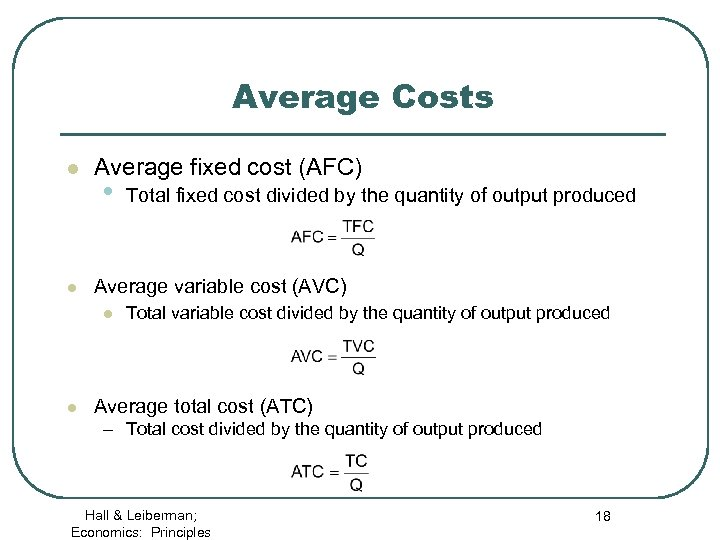Average Costs l l Average fixed cost (AFC) • Average variable cost (AVC) l l Total fixed cost divided by the quantity of output produced Total variable cost divided by the quantity of output produced Average total cost (ATC) – Total cost divided by the quantity of output produced Hall & Leiberman; Economics: Principles 18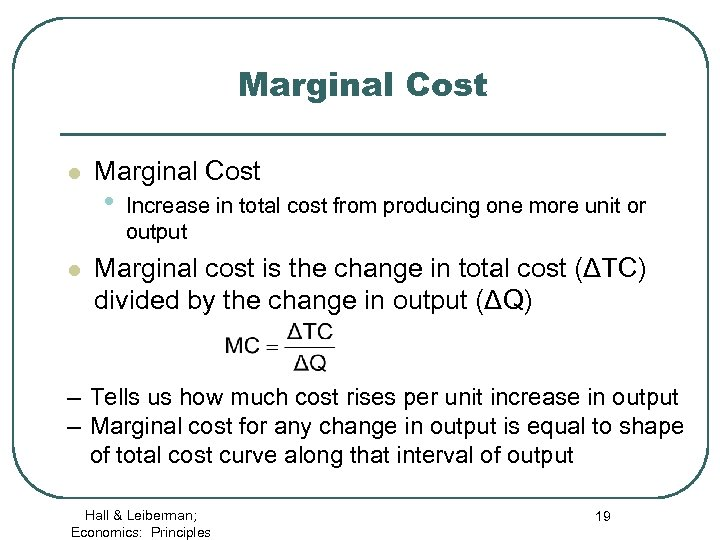Marginal Cost l l Marginal Cost • Increase in total cost from producing one more unit or output Marginal cost is the change in total cost (ΔTC) divided by the change in output (ΔQ) – Tells us how much cost rises per unit increase in output – Marginal cost for any change in output is equal to shape of total cost curve along that interval of output Hall & Leiberman; Economics: Principles 19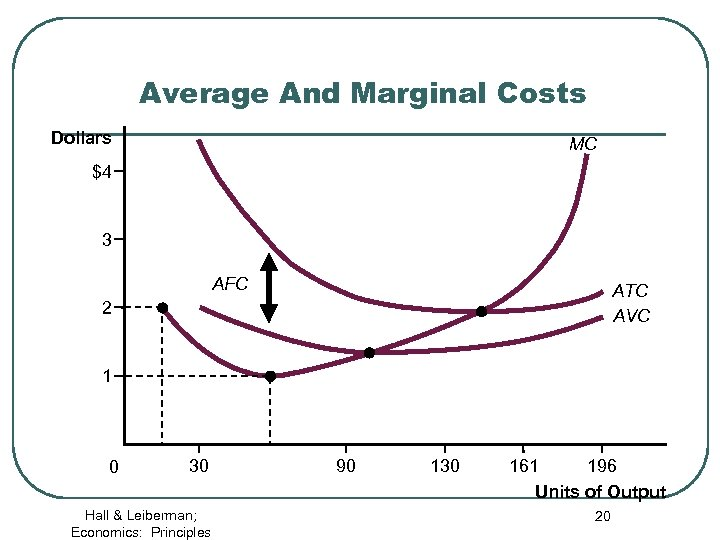Average And Marginal Costs Dollars MC \$4 3 AFC ATC AVC 2 1 0 30 Hall & Leiberman; Economics: Principles 90 130 161 196 Units of Output 20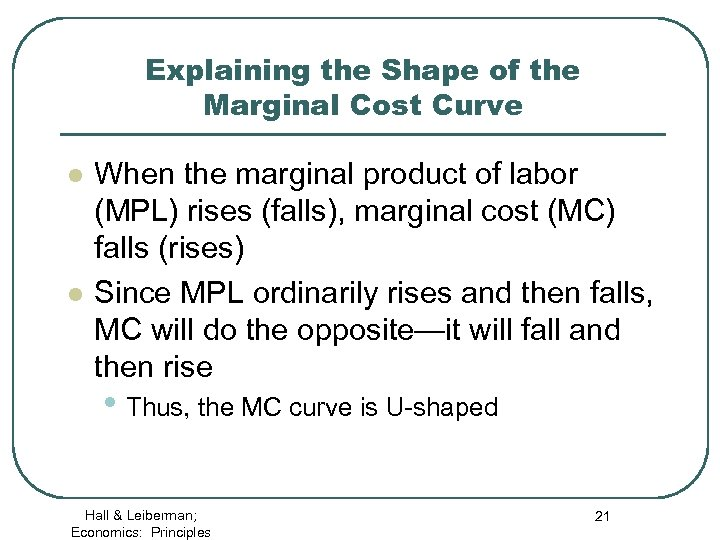Explaining the Shape of the Marginal Cost Curve l l When the marginal product of labor (MPL) rises (falls), marginal cost (MC) falls (rises) Since MPL ordinarily rises and then falls, MC will do the opposite—it will fall and then rise • Thus, the MC curve is U-shaped Hall & Leiberman; Economics: Principles 21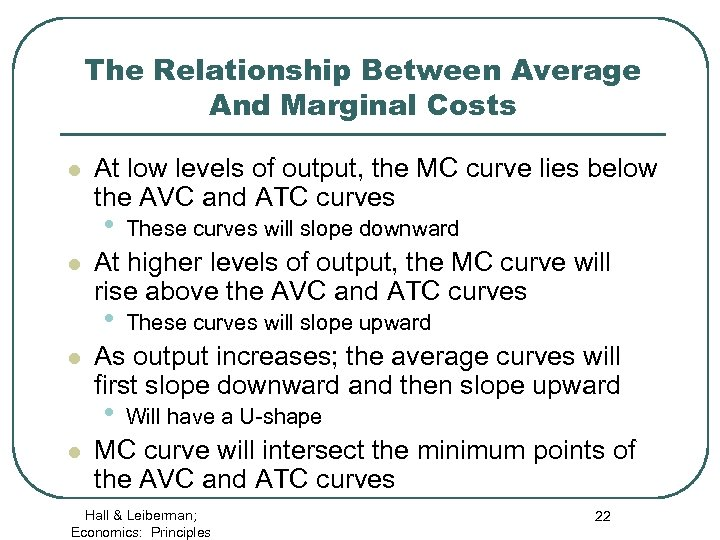The Relationship Between Average And Marginal Costs l At low levels of output, the MC curve lies below the AVC and ATC curves • l At higher levels of output, the MC curve will rise above the AVC and ATC curves • l These curves will slope upward As output increases; the average curves will first slope downward and then slope upward • l These curves will slope downward Will have a U-shape MC curve will intersect the minimum points of the AVC and ATC curves Hall & Leiberman; Economics: Principles 22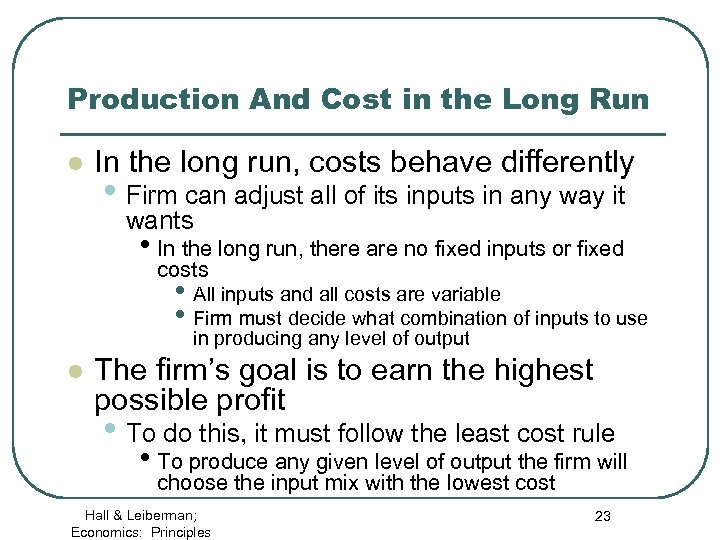Production And Cost in the Long Run l In the long run, costs behave differently • Firm can adjust all of its inputs in any way it wants • In the long run, there are no fixed inputs or fixed costs • All inputs and all costs are variable • Firm must decide what combination of inputs to use in producing any level of output l The firm’s goal is to earn the highest possible profit • To do this, it must follow the least cost rule • To produce any given level of output the firm will choose the input mix with the lowest cost Hall & Leiberman; Economics: Principles 23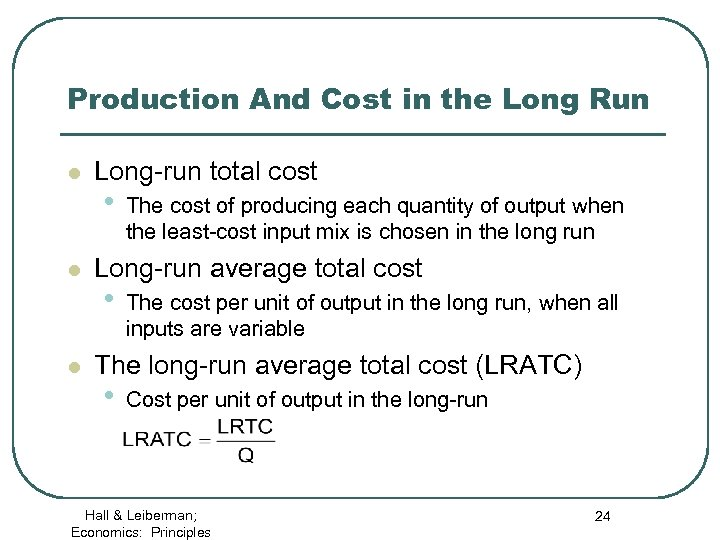Production And Cost in the Long Run l l l Long-run total cost • The cost of producing each quantity of output when the least-cost input mix is chosen in the long run Long-run average total cost • The cost per unit of output in the long run, when all inputs are variable The long-run average total cost (LRATC) • Cost per unit of output in the long-run Hall & Leiberman; Economics: Principles 24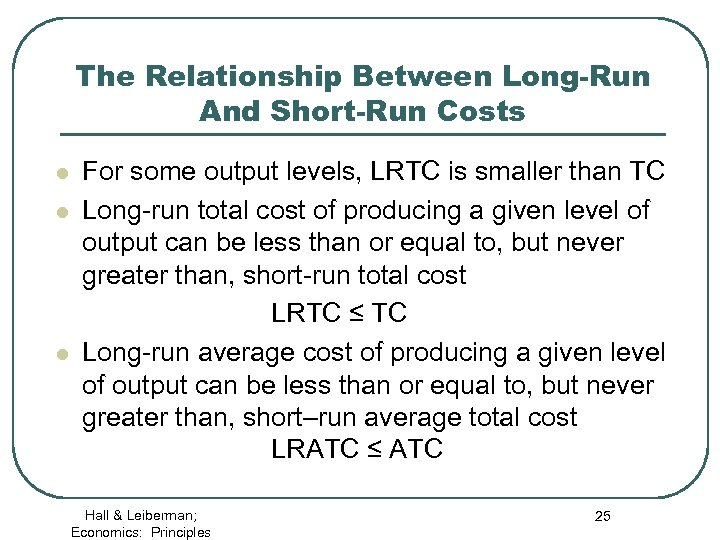The Relationship Between Long-Run And Short-Run Costs l l l For some output levels, LRTC is smaller than TC Long-run total cost of producing a given level of output can be less than or equal to, but never greater than, short-run total cost LRTC ≤ TC Long-run average cost of producing a given level of output can be less than or equal to, but never greater than, short–run average total cost LRATC ≤ ATC Hall & Leiberman; Economics: Principles 25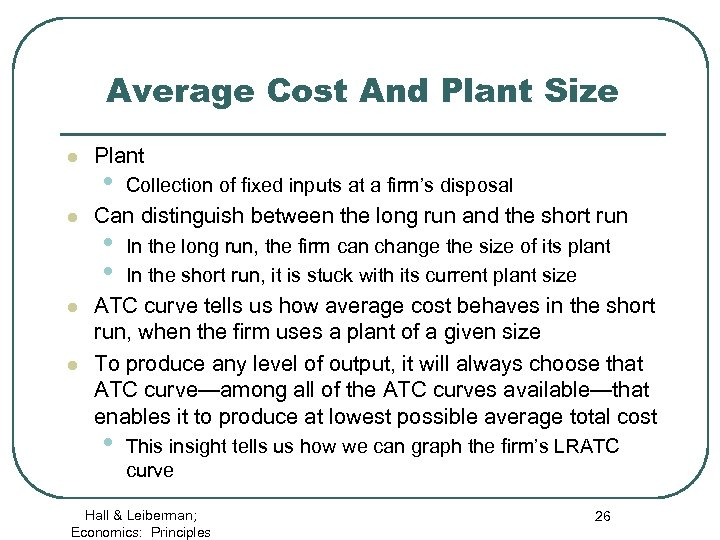Average Cost And Plant Size l l Plant • Collection of fixed inputs at a firm’s disposal Can distinguish between the long run and the short run • • In the long run, the firm can change the size of its plant In the short run, it is stuck with its current plant size ATC curve tells us how average cost behaves in the short run, when the firm uses a plant of a given size To produce any level of output, it will always choose that ATC curve—among all of the ATC curves available—that enables it to produce at lowest possible average total cost • This insight tells us how we can graph the firm’s LRATC curve Hall & Leiberman; Economics: Principles 26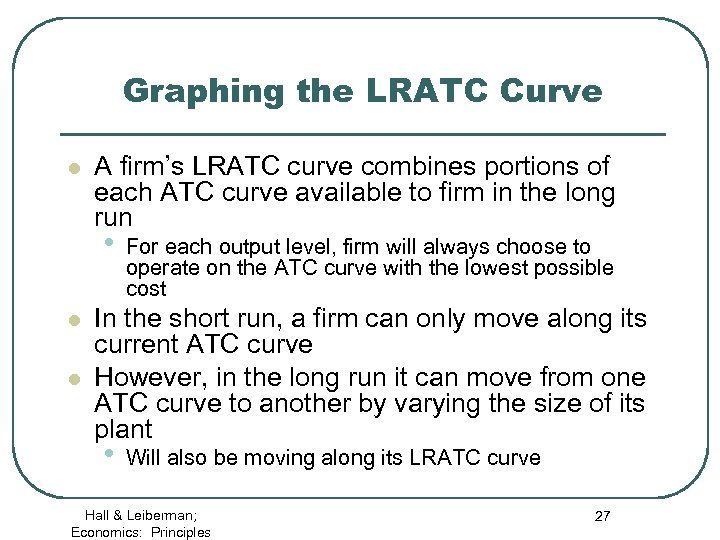Graphing the LRATC Curve l A firm’s LRATC curve combines portions of each ATC curve available to firm in the long run • l l For each output level, firm will always choose to operate on the ATC curve with the lowest possible cost In the short run, a firm can only move along its current ATC curve However, in the long run it can move from one ATC curve to another by varying the size of its plant • Will also be moving along its LRATC curve Hall & Leiberman; Economics: Principles 27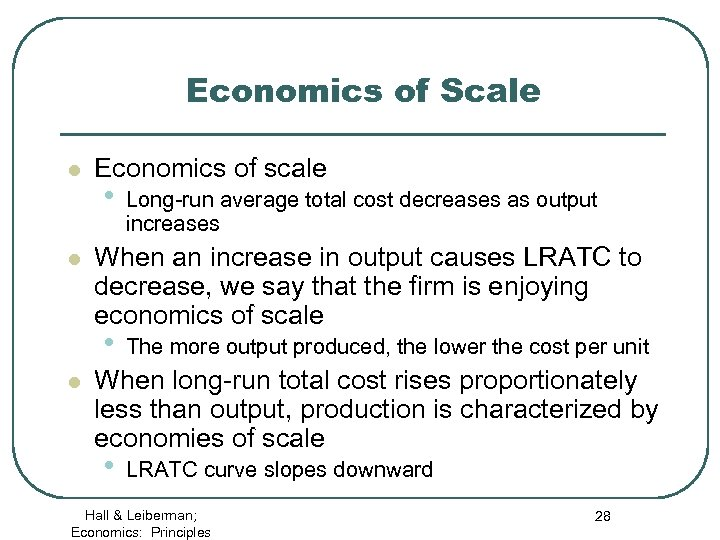Economics of Scale l l Economics of scale • When an increase in output causes LRATC to decrease, we say that the firm is enjoying economics of scale • l Long-run average total cost decreases as output increases The more output produced, the lower the cost per unit When long-run total cost rises proportionately less than output, production is characterized by economies of scale • LRATC curve slopes downward Hall & Leiberman; Economics: Principles 28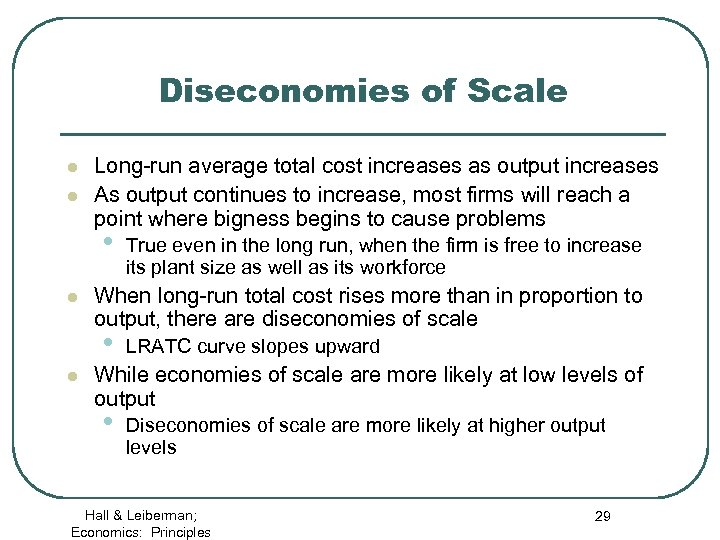Diseconomies of Scale l l Long-run average total cost increases as output increases As output continues to increase, most firms will reach a point where bigness begins to cause problems • l When long-run total cost rises more than in proportion to output, there are diseconomies of scale • l True even in the long run, when the firm is free to increase its plant size as well as its workforce LRATC curve slopes upward While economies of scale are more likely at low levels of output • Diseconomies of scale are more likely at higher output levels Hall & Leiberman; Economics: Principles 29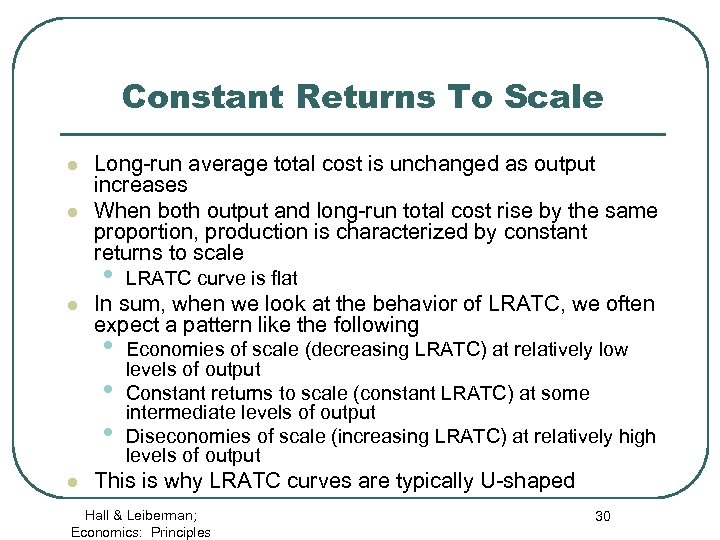Constant Returns To Scale l l Long-run average total cost is unchanged as output increases When both output and long-run total cost rise by the same proportion, production is characterized by constant returns to scale • • l l LRATC curve is flat Economies of scale (decreasing LRATC) at relatively low levels of output Constant returns to scale (constant LRATC) at some intermediate levels of output Diseconomies of scale (increasing LRATC) at relatively high levels of output In sum, when we look at the behavior of LRATC, we often expect a pattern like the following This is why LRATC curves are typically U-shaped Hall & Leiberman; Economics: Principles 30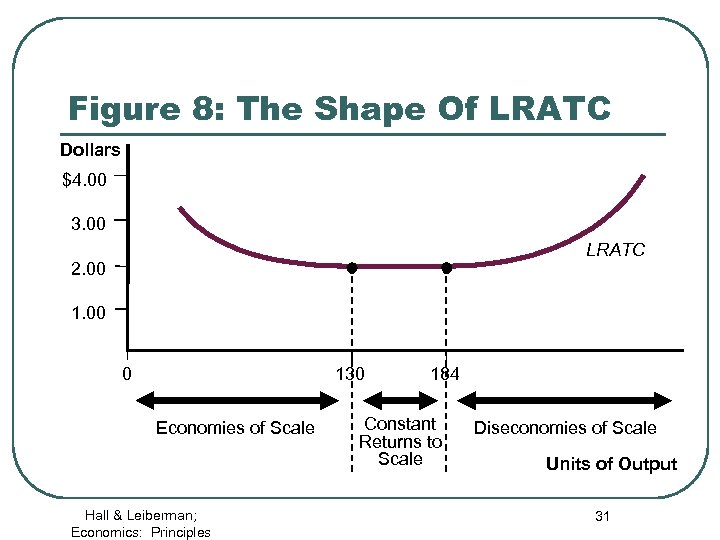Figure 8: The Shape Of LRATC Dollars \$4. 00 3. 00 LRATC 2. 00 130 0 Economies of Scale Hall & Leiberman; Economics: Principles 184 Constant Returns to Scale Diseconomies of Scale Units of Output 31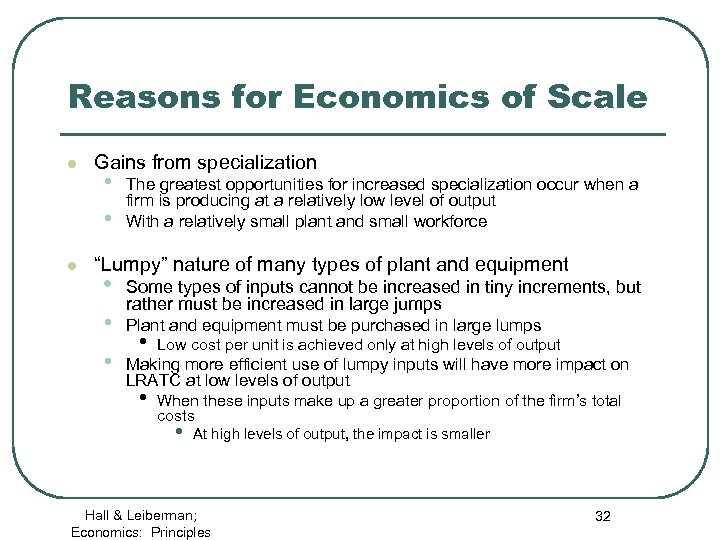Reasons for Economics of Scale l l Gains from specialization • • The greatest opportunities for increased specialization occur when a firm is producing at a relatively low level of output With a relatively small plant and small workforce “Lumpy” nature of many types of plant and equipment • • Some types of inputs cannot be increased in tiny increments, but rather must be increased in large jumps Plant and equipment must be purchased in large lumps Low cost per unit is achieved only at high levels of output • • • When these inputs make up a greater proportion of the firm’s total costs Making more efficient use of lumpy inputs will have more impact on LRATC at low levels of output • At high levels of output, the impact is smaller Hall & Leiberman; Economics: Principles 32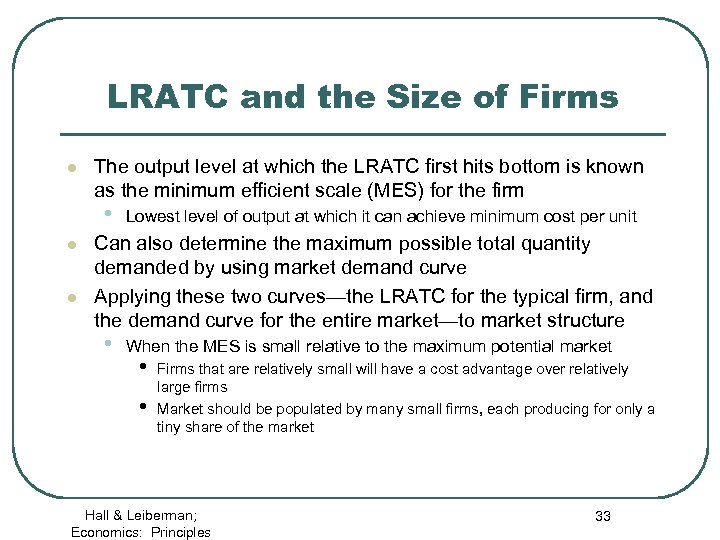LRATC and the Size of Firms l The output level at which the LRATC first hits bottom is known as the minimum efficient scale (MES) for the firm • l l Lowest level of output at which it can achieve minimum cost per unit Can also determine the maximum possible total quantity demanded by using market demand curve Applying these two curves—the LRATC for the typical firm, and the demand curve for the entire market—to market structure • When the MES is small relative to the maximum potential market • • Firms that are relatively small will have a cost advantage over relatively large firms Market should be populated by many small firms, each producing for only a tiny share of the market Hall & Leiberman; Economics: Principles 33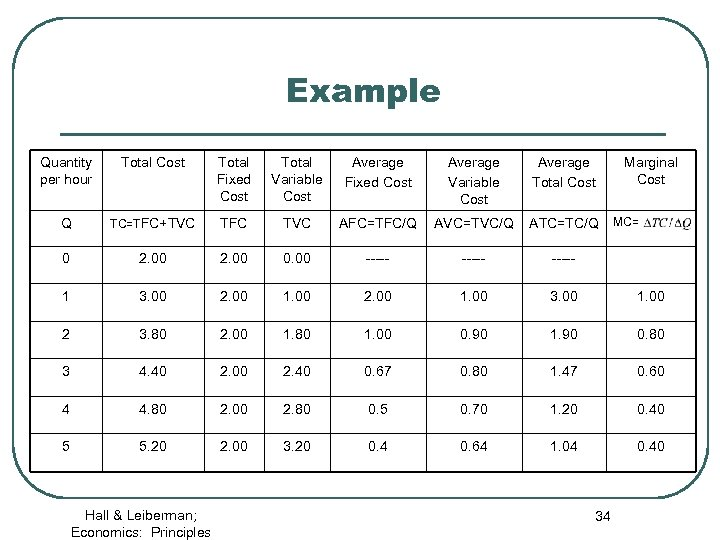Example Quantity per hour Total Cost Total Fixed Cost Total Variable Cost Average Fixed Cost Average Variable Cost Average Total Cost Q TC=TFC+TVC TFC TVC AFC=TFC/Q AVC=TVC/Q ATC=TC/Q 0 2. 00 0. 00 ----- 1 3. 00 2. 00 1. 00 3. 00 1. 00 2 3. 80 2. 00 1. 80 1. 00 0. 90 1. 90 0. 80 3 4. 40 2. 00 2. 40 0. 67 0. 80 1. 47 0. 60 4 4. 80 2. 00 2. 80 0. 5 0. 70 1. 20 0. 40 5 5. 20 2. 00 3. 20 0. 4 0. 64 1. 04 0. 40 Hall & Leiberman; Economics: Principles 34 Marginal Cost MC=